# Logical Reasoning - Statement and Conclusion

>>>>>>>>Statement and Conclusion

In each of the questions below, two statements are followed by the two conclusions I and II. Assuming that the given statements are true even if they are at variance with the commonly known facts, pick one of the following answers that you think is correct.

• A

only conclusion I follows.• B

only conclusion II follows.• C

both I and II follows.• D

neither I nor II follows.• Option : D
• Explanation : Step I: First, we check that there are three terms, tables, golden and teak. Here, table is the middle term. Then, we see whether it is in the form: A to B and B to C. It is not; so alignment is required. For alignment, we just need to change the order in this case.
After alignment:
All teak are tables.
Some tables are golden.
Step II: This is ‘A + I’. (Please refer to ‘syllogism rule table’. No conclusion can be drawn.
So, option (d) is the answer.
Venn diagram solution: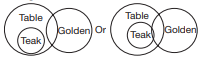Conclusion I: Some teak may or may not be golden. There is ambiguity. Conclusion I does not follow.
Conclusion II: Similarly, some golden may or may not be teak.
As there is ambiguity in both cases, none follows. Thus, option (d) is the answer.

• A

only conclusion I follows.• B

only conclusion II follows.• C

both I and II follows.• D

neither I nor II follows.• Option : A
• Explanation : First check the number of terms. It is three. Now look at alignment.
Now, the common term ‘man’ is not in the form A to B and B to C. So alignment is required.
Align this pair as ‘Karan is a man’ and ‘No man is a Tiger’. Here, it is important to mention that statement ‘Karan is a Tiger’ is considered as A type.
Now, A + E = E. So conclusion I ‘Karan is not a Tiger’ follows.
Alternative solution:
Venn diagram solution: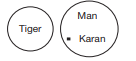• A

only conclusion I follows.• B

only conclusion II follows.• C

both I and II follows.• D

neither I nor II follows.• Option : C
• Explanation : There are three terms. No alignment is required as it is in the standard format.
A + A = A. The conclusion is ‘all boys are fathers’. Conclusion II follows.
By applying immediate reference to (converting) statement I, we can say that some men are boys. Conclusion I also follows. Both I and II follows.
Venn diagram solution: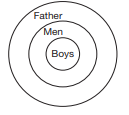• A

only conclusion I follows.• B

only conclusion II follows.• C

both I and II follows.• D

neither I nor II follows.• Option : B
• Explanation : Three terms—Teacher, girls, and dull. Next, it is in proper format. No alignment is required.
Now, A + E = E. That is ‘No Teacher is dull’. Conclusion II follows. Boys is not mentioned as a term. So nothing can be said about conclusion I.
Venn diagram solution: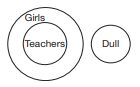• A

only conclusion I follows.• B

only conclusion II follows.• C

both I and II follows.• D

neither I nor II follows.• Option : D
• Explanation : There are three terms—poets, inspiring, and artists.
All poets (A) are inspiring (B).
All artists (C) are inspiring (B).
A to B.
C to B.
So alignment is required as it should be in the form A to B and B to C.
After converting second statement.
All poets are inspiring (A type).
Some inspiring persons are artists (I type). It contradicts second conclusion also.
A + I = No conclusion.
So, option (d) is the answer.
Venn diagram solution: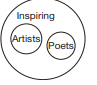## Description

• Statements And Conclusions Questions can be used to give quizzes by any candidate who is preparing for UGC NET Paper 1
• This Statements And Conclusions Questions section will help you test your analytical skills in a tricky method, thereby giving you an edge over other students
• All candidates who have to appear for the Kendriya Vidyalaya Entrance exam can also refer to this mcq section.
• You can also get access to the Statements And Conclusions MCQ ebook.
• Statements And Conclusions Questions can be used in the preparation of JRF, CSIR, and various other exams.